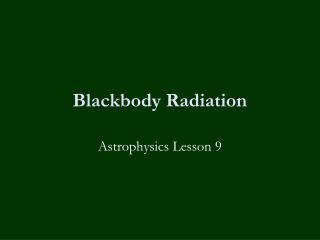Download PresentationBlackbody Radiation

# Blackbody Radiation

Download Presentation## Blackbody Radiation

- - - - - - - - - - - - - - - - - - - - - - - - - - - E N D - - - - - - - - - - - - - - - - - - - - - - - - - - -
##### Presentation Transcript

1. Blackbody Radiation Astrophysics Lesson 9

2. Homework None today, module exams?

3. Learning Objectives Define the term blackbody. Sketch and describe how shape of black body curves change as temperature is increased. Use Wien’s displacement law to estimate black-body temperature of sources. Use Stefan’s law to estimate area needed for sources to have same power output as the sun. Recap inverse square law, state assumptions in its application.

4. Definition of a Blackbody • A body that absorbs all wavelengths of electromagnetic radiation and can emit all wavelengths of electromagnetic radiation. Pure black surfaces emit radiation strongly and in a well-defined way – this is called blackbody radiation. It is a reasonable approximation to assume that stars behave as black bodies.

5. Black Body Radiation • The blackbody radiation of stars produces a continuous spectrum. • A graph of intensity against wavelength for black body radiation is known as a black body curve. • The blackbody curve is dependent on the temperature.

6. Black Body Curve Shape

7. Note the following for black bodies: • a hot object emits radiation across a wide range of wavelength; As the temperature of the object increases:- • peak of the graph moves towards the shorter wavelengths. • the peak is higher • the area under the graph is the total energy radiated per unit time per unit surface area.

8. Wien’s Displacement Law • The peak wavelength, λmax, is the wavelength at which maximum energy is radiated.  • This is inversely proportional to the temperature, T, in Kelvin. • This is called Wien's  Displacement Law (as the peak is displaced towards shorter wavelengths):- • Note the units of mK means a metrekelvin.

9. Worked Example • What is the peak wavelength of a black body emitting radiation at 2000 K?  In what part of the electromagnetic spectrum does this lie? • λmax = 0.0029 mK ÷ 2000 K • λmax = 1.45 x 10-6 m = 1450 nm • This is in the infra-red region.

10. Question • Betelgeuse appears to be red.  If red light has a wavelength of about 600 nm, what would the surface temperature be? • Why no green stars?

11. Answer • Betelgeuse appears to be red.  If red light has a wavelength of about 600 nm, what would the surface temperature be? • T = 0.0029 mK ÷ 600 x 10-9 m  • T = 4800 K • Why no green stars?

12. Why no green stars? • You don't get green stars because the light from stars is emitted at a range of wavelengths, so there is mixing of colours.  So those stars with a λmax in the green region will actually appear to be white.

13. Luminosity of Stars • The luminosity of a star is the total energy given out per second, so it's the power.  • From the graph the luminosity increases rapidly with temperature, which gives rise to Stefan's Law.  • The total energy per unit time radiated by a black body is proportional to the fourth power of its absolute temperature.

14. Stefan’s Law • In other words double the temperature and the power goes up sixteen times.  In symbols: • L – Luminosity of the star (W) • σ – Stefan's constant = 5.67 x 10-8 W m-2 K-4 • A – surface area (m2) • T – surface temperature (K)

15. Applied to Stars • We can treat a star as a perfect sphere (A = 4πr2) and a perfect black body.  So for any star, radius r, we can write:

16. Inverse Square Law • From Earth we can measure the intensity of the star:- Where L is the luminosity of the star d is the distance from the star

17. Question • If the Sun has a radius of 6.96 x 108 m and a surface temperature of about 6000 K, what is its total power output?  • What is the power per unit area?  • What is the peak wavelength?

18. Answer • L = 4 ×π× (6.96 x 108 m)2× 5.67 × 10-8 W m-2 K-4 x (6000 K)4 • L = 4.47 × 1026 W • The power per unit area = 4.47 × 1026 W ÷ 6.09 × 1018 m2 = 7.34 × 107  W/m2 • Peak wavelength λmax = 0.0029 mK ÷ 6000 K = 4.82 × 10-7 m = 482 nm